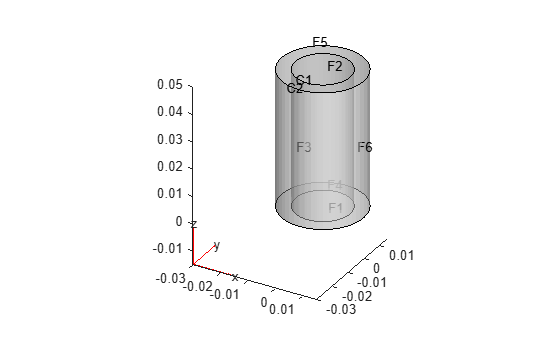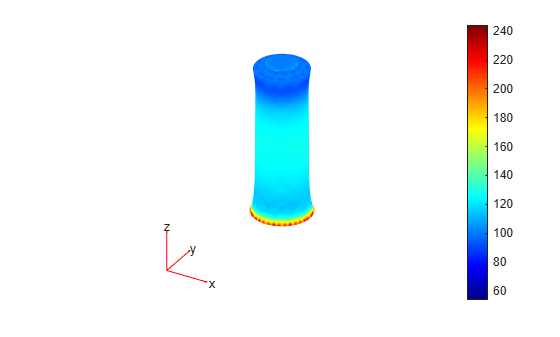Documentation

# StaticStructuralResults

Static structural solution and its derived quantities

## Description

A `StaticStructuralResults` object contains the displacement, stress, strain, and von Mises stress in a form convenient for plotting and postprocessing.

Displacements, stresses, and strains are reported for the nodes of the triangular or tetrahedral mesh generated by `generateMesh`. Displacement values at the nodes appear as a structure array in the `Displacement` property. The fields of the structure array contain components of displacement at nodal locations.

Stress and strain values at the nodes appear as structure arrays in the `Stress` and `Strain` properties, respectively.

von Mises stress at the nodes appears as a vector in the `VonMisesStress` property.

To interpolate the displacement, stress, strain, and von Mises stress to a custom grid, such as the one specified by `meshgrid`, use `interpolateDisplacement`, `interpolateStress`, `interpolateStrain`, and `interpolateVonMisesStress`, respectively.

To evaluate reaction forces on a specified boundary, use `evaluateReaction`. To evaluate principal stress and principal strain at nodal locations, use `evaluatePrincipalStress` and `evaluatePrincipalStrain`, respectively.

## Creation

Solve a static linear elasticity problem by using the `solve` function. This function returns a static structural solution as a `StaticStructuralResults` object.

## Properties

expand all

Displacement values at the nodes, returned as a structure array. The fields of the structure array contain components of displacement at nodal locations.

Data Types: `struct`

Stress values at the nodes, returned as a structure array. The fields of the structure array contain components of stress at nodal locations.

Data Types: `struct`

Strain values at the nodes, returned as a structure array. The fields of the structure array contain components of strain at nodal locations.

Data Types: `struct`

Von Mises stress values at the nodes, returned as a vector.

Data Types: `struct`

Finite element mesh, returned as a `FEMesh` object. For details, see FEMesh Properties.

## Object Functions

 `interpolateDisplacement` Interpolate displacement at arbitrary spatial locations `interpolateStress` Interpolate stress at arbitrary spatial locations `interpolateStrain` Interpolate strain at arbitrary spatial locations `interpolateVonMisesStress` Interpolate von Mises stress at arbitrary spatial locations `evaluateReaction` Evaluate reaction forces on boundary `evaluatePrincipalStress` Evaluate principal stress at nodal locations `evaluatePrincipalStrain` Evaluate principal strain at nodal locations

## Examples

collapse all

Solve a static structural model representing a bimetallic cable under tension.

Create a static structural model for solving a solid (3-D) problem.

`structuralmodel = createpde('structural','static-solid');`

Create the geometry and include it in the model. Plot the geometry.

```gm = multicylinder([0.01,0.015],0.05); structuralmodel.Geometry = gm; pdegplot(structuralmodel,'FaceLabels','on','CellLabels','on','FaceAlpha',0.5)```Specify the Young's modulus and Poisson's ratio for each metal.

```structuralProperties(structuralmodel,'Cell',1,'YoungsModulus',110E9, ... 'PoissonsRatio',0.28); structuralProperties(structuralmodel,'Cell',2,'YoungsModulus',210E9, ... 'PoissonsRatio',0.3);```

Specify that faces 1 and 4 are fixed boundaries.

`structuralBC(structuralmodel,'Face',[1,4],'Constraint','fixed');`

Specify the surface traction for faces 2 and 5.

`structuralBoundaryLoad(structuralmodel,'Face',[2,5],'SurfaceTraction',[0;0;100]);`

Generate a mesh and solve the problem.

```generateMesh(structuralmodel); structuralresults = solve(structuralmodel)```
```structuralresults = StaticStructuralResults with properties: Displacement: [1x1 struct] Strain: [1x1 struct] Stress: [1x1 struct] VonMisesStress: [22281x1 double] Mesh: [1x1 FEMesh] ```

The solver finds the values of displacement, stress, strain, and von Mises stress at the nodal locations. To access these values, use `structuralresults.Displacement`, `structuralresults.Stress`, and so on. The displacement, stress, and strain values at the nodal locations are returned as structure arrays with fields representing their components.

`structuralresults.Displacement`
```ans = struct with fields: ux: [22281x1 double] uy: [22281x1 double] uz: [22281x1 double] Magnitude: [22281x1 double] ```
`structuralresults.Stress`
```ans = struct with fields: sxx: [22281x1 double] syy: [22281x1 double] szz: [22281x1 double] syz: [22281x1 double] sxz: [22281x1 double] sxy: [22281x1 double] ```
`structuralresults.Strain`
```ans = struct with fields: exx: [22281x1 double] eyy: [22281x1 double] ezz: [22281x1 double] eyz: [22281x1 double] exz: [22281x1 double] exy: [22281x1 double] ```

Plot the deformed shape with the z-component of normal stress.

```pdeplot3D(structuralmodel,'ColorMapData',structuralresults.Stress.szz, ... 'Deformation',structuralresults.Displacement)```